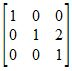# How to Solve Inverse Matrices

Instructions:

Choose an answer and hit 'next'. You will receive your score and answers at the end.

question 1 of 3

### The identity matrix can be likened to what number?

Create Your Account To Take This Quiz

As a member, you'll also get unlimited access to over 75,000 lessons in math, English, science, history, and more. Plus, get practice tests, quizzes, and personalized coaching to help you succeed.

Try it risk-free for 30 days. Cancel anytime.

### 1. Which number needs to be changed to find the inverse of this matrix?### 2. A matrix multiplied by its inverse is equal to what?

Create your account to access this entire worksheet
A Premium account gives you access to all lesson, practice exams, quizzes & worksheets
Quizzes, practice exams & worksheets
Certificate of Completion
Create an account to get started

This quiz/worksheet combination will test your understanding of identity and inverse matrices. Questions will cover general information about inverse matrices and how to find inverse matrices by multiplying.

## Quiz and Worksheet Goals

In this assessment, you will be tested on:

• Which number the inverse matrix can be likened to
• Which rows of a matrix to use to find an inverse matrix
• How to use identity matrices to solve matrix equations
• Which numbers to use to find the inverse
• The result of multiplying a matrix by its identity matrix

## Skills Practiced

• Information Recall: access the information you've gained regarding identity and inverse matrices
• Problem Solving: use acquired knowledge to solve inverse matrix practice problems
• Knowledge Application: use your knowledge to answer questions about multiplying identity and inverse matrices

To learn more about solving inverse matrices, review the accompanying lesson. This lesson covers the following objectives:

• Define inverse and identity matrices
• Know how to find the inverse of a particular matrix
• Understand how to perform matrix operations
• Solve equations that involve matrices
Final ExamAlgebra II Textbook
Status: Not Started
Chapter ExamAlgebra II: Matrices and Determinants
Status: Not Started

Support